# RS Aggarwal Solutions for Class 8 Maths Chapter 11 - Compound Interest

RS Aggarwal Solutions For Class 8 Maths Chapter 11 Compound Interest is provided here. You can download the pdf of RS Aggarwal Solutions for Class 8 Maths Chapter 11 Compound Interest from the given links. RS Aggarwal Solutions helps students to attain a good score in the examinations, while also providing extensive knowledge about the subject, as Class 8 is a critical stage in their academic career. Therefore, we at BYJU’S provide answers to all questions uniquely and briefly.

RS Aggarwal Solutions For Class 8 Maths also provides the foundation to higher studies. RS Aggarwal Solutions For Class 8 Maths Chapter 11 Compound Interest provides the concepts in an effective way.Here we will learn about different methods of calculating the Compound Interest.

## Download pdf of RS Aggarwal Solutions For Class 8 Maths Chapter 11 Compound Interest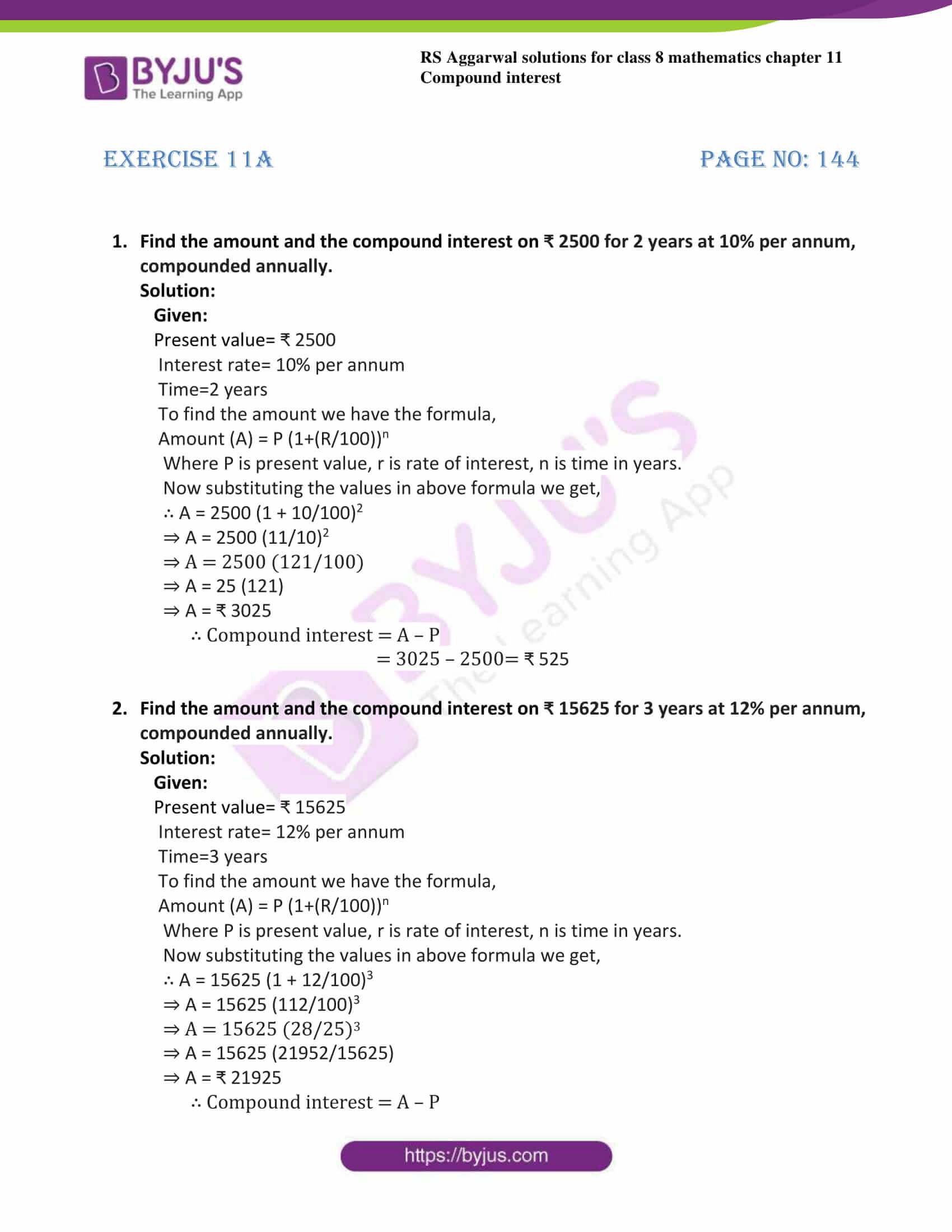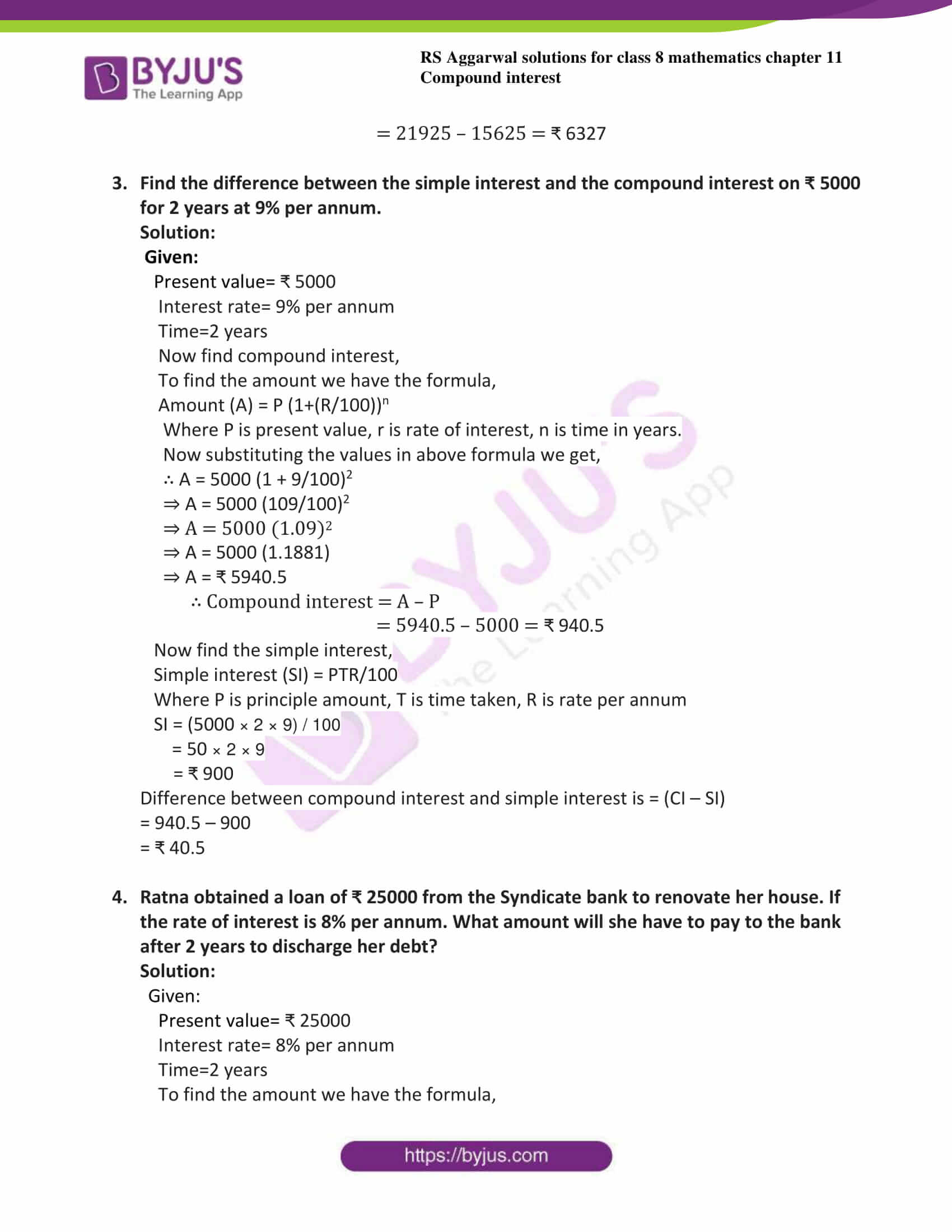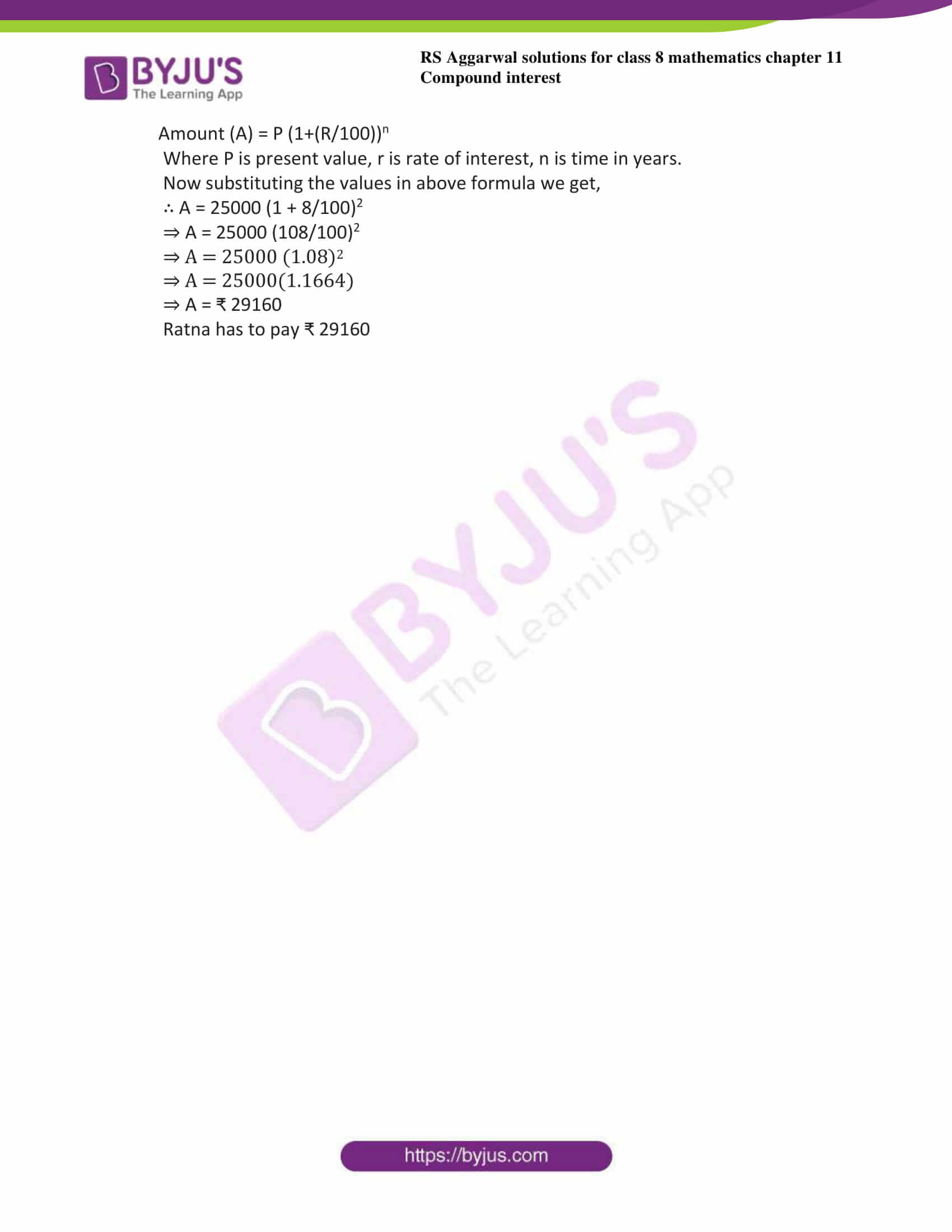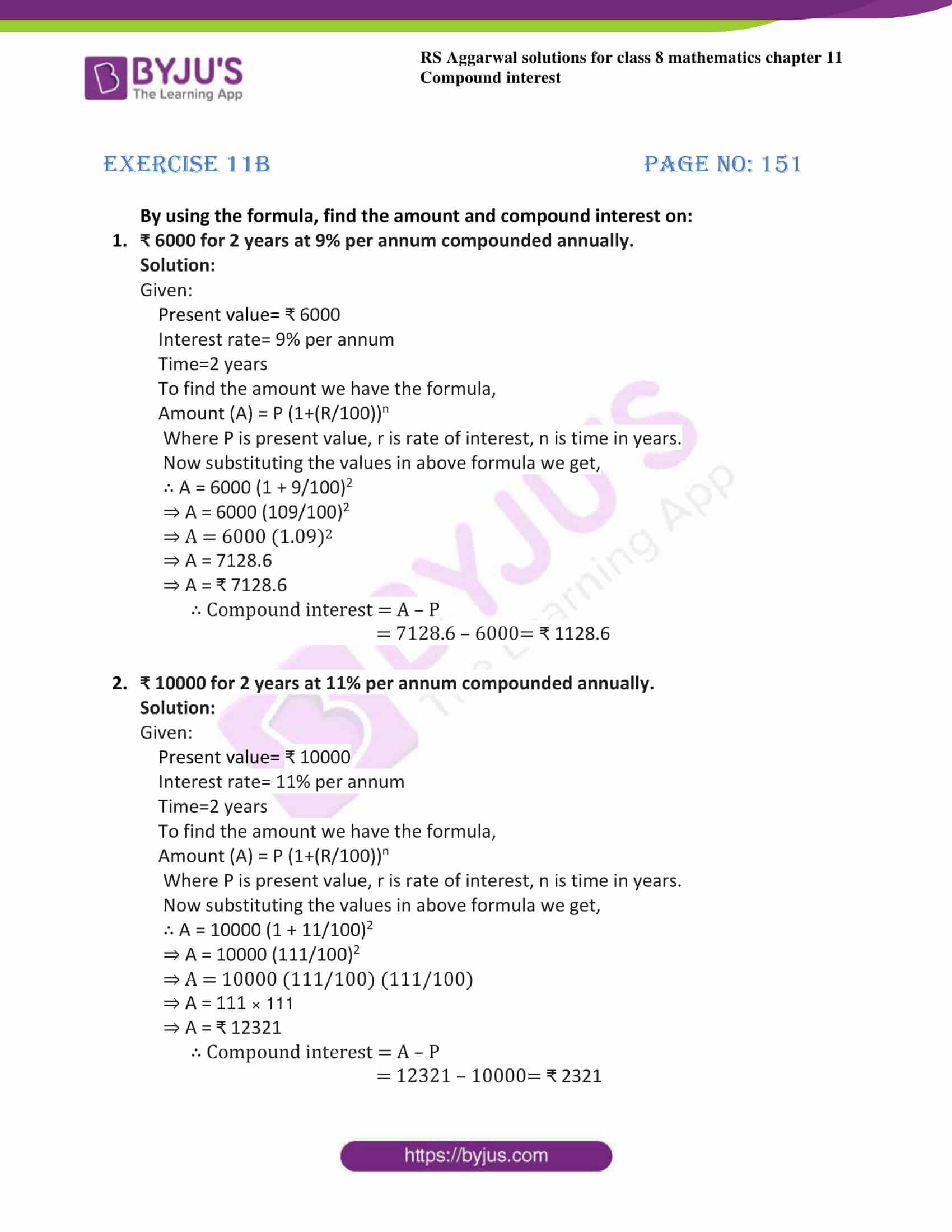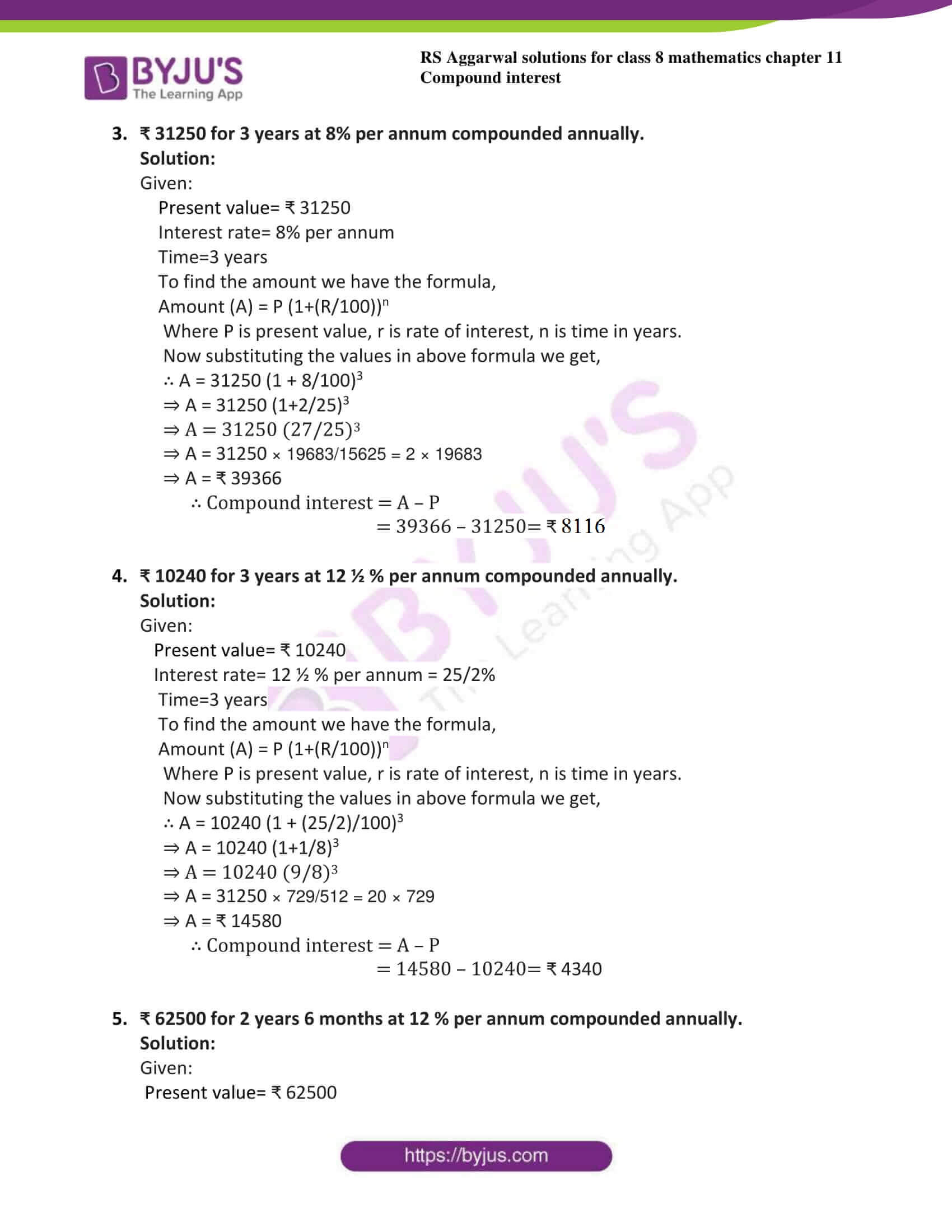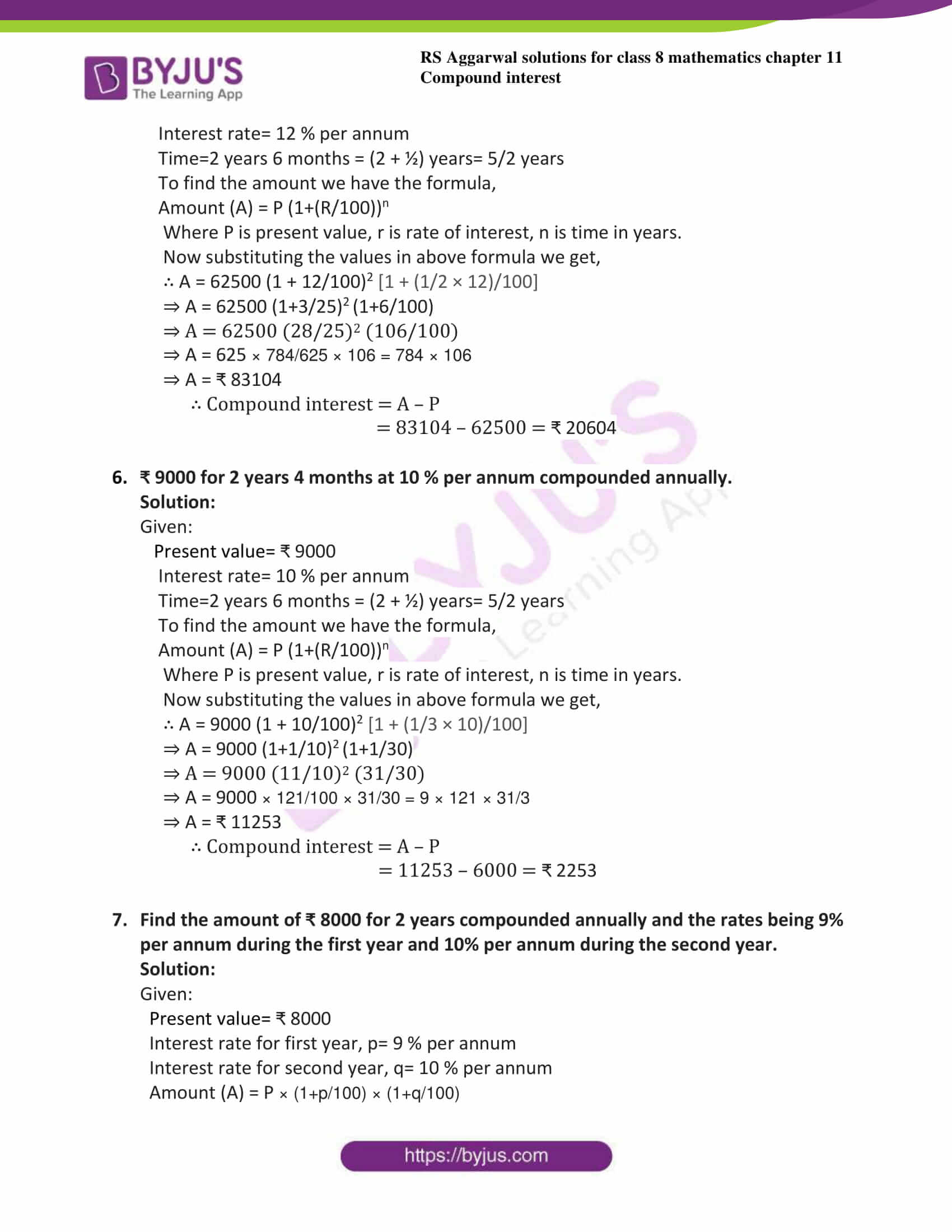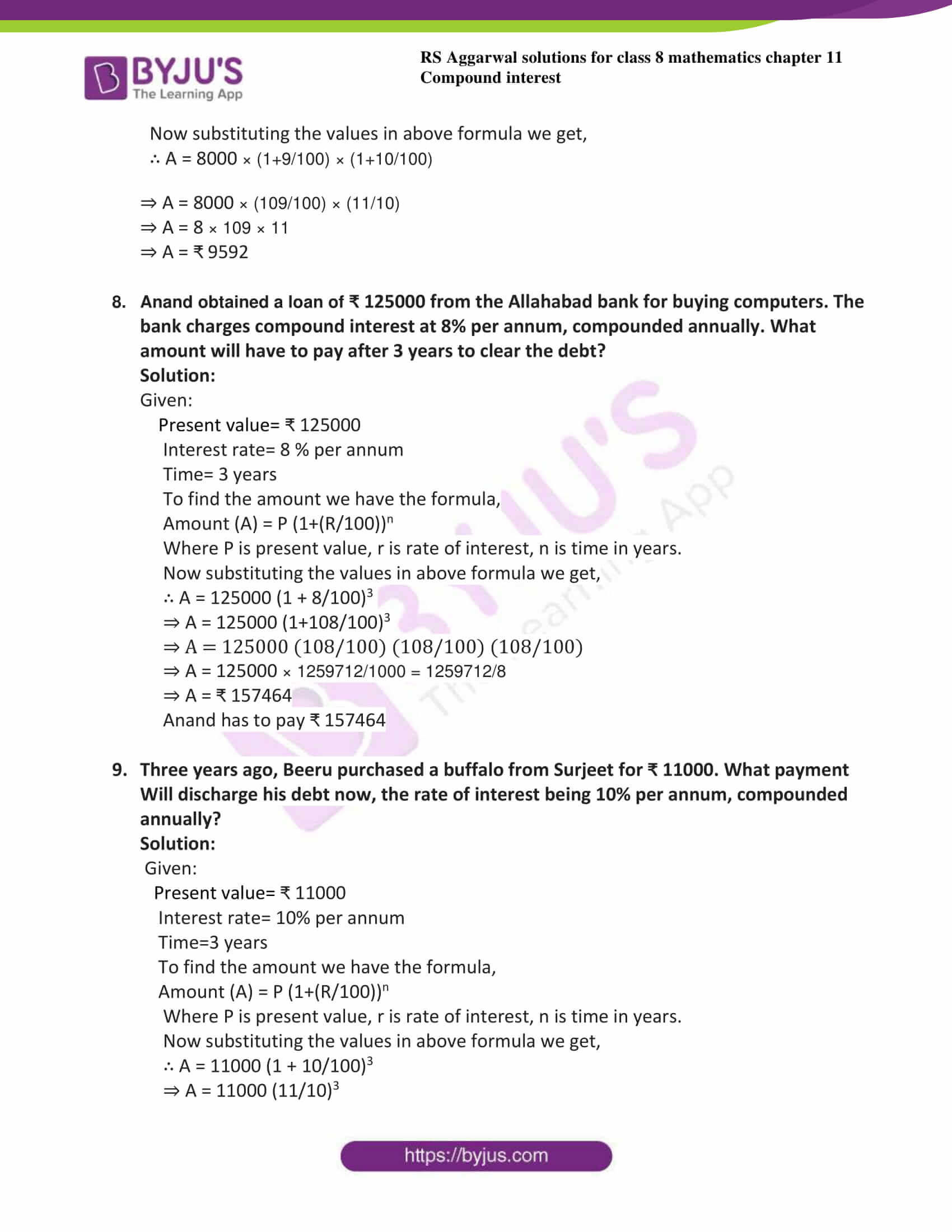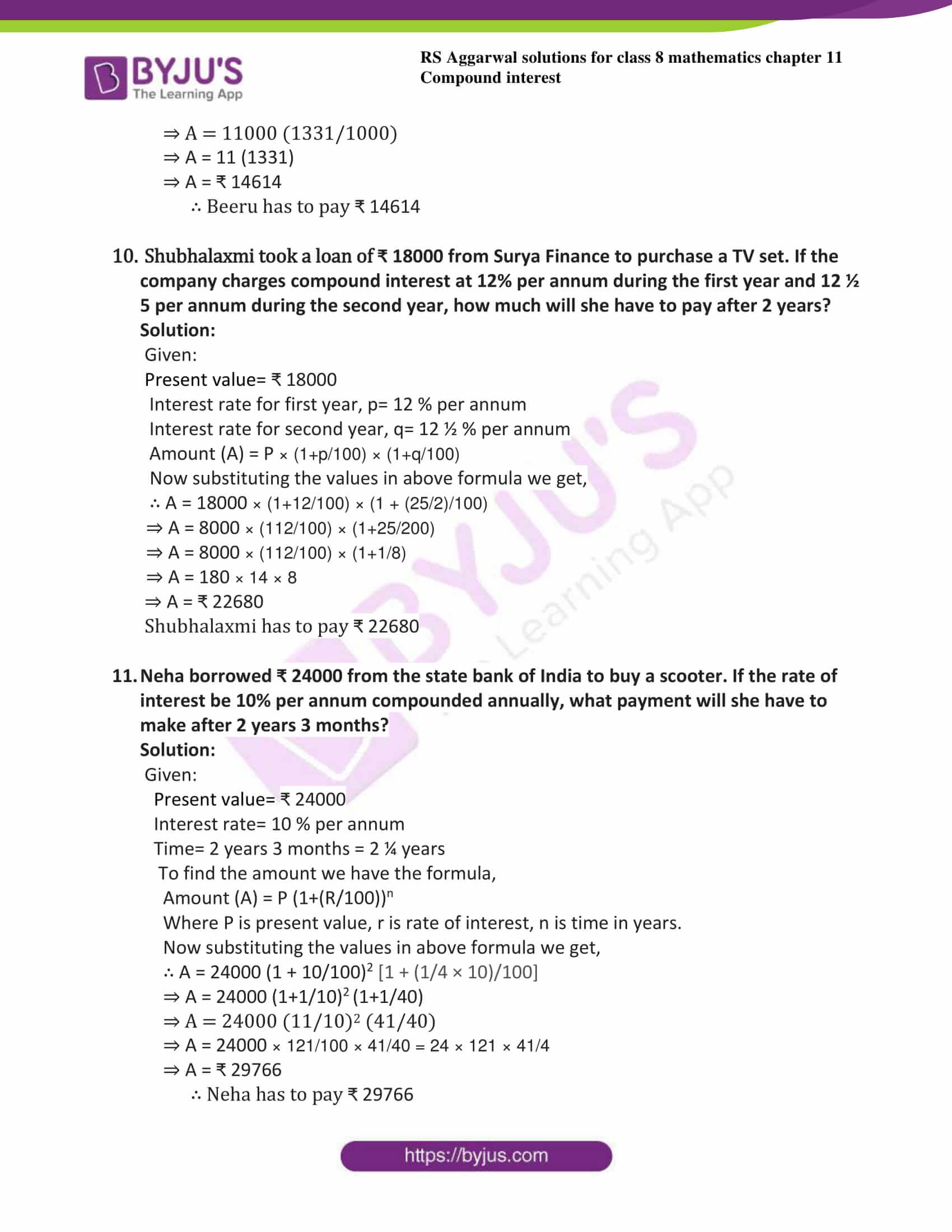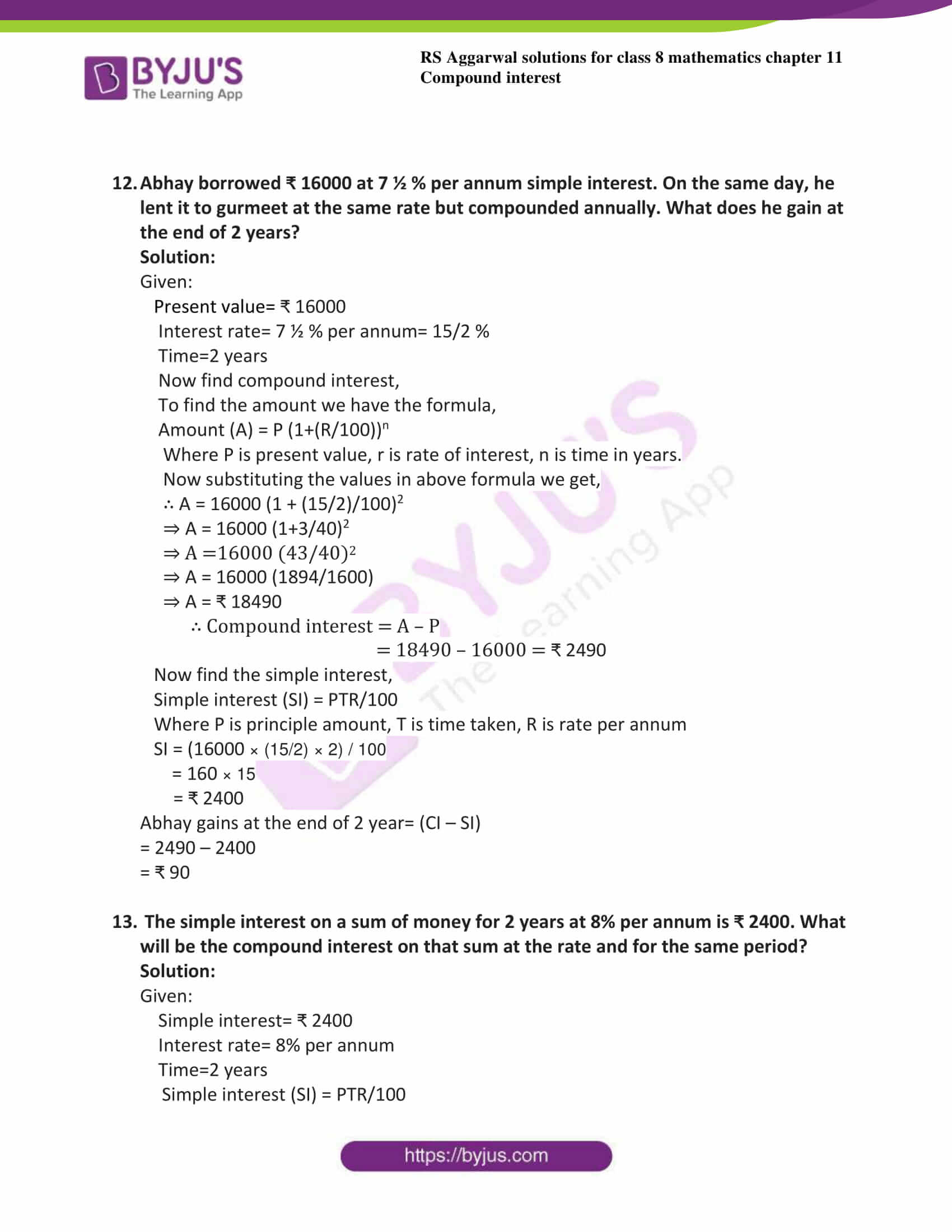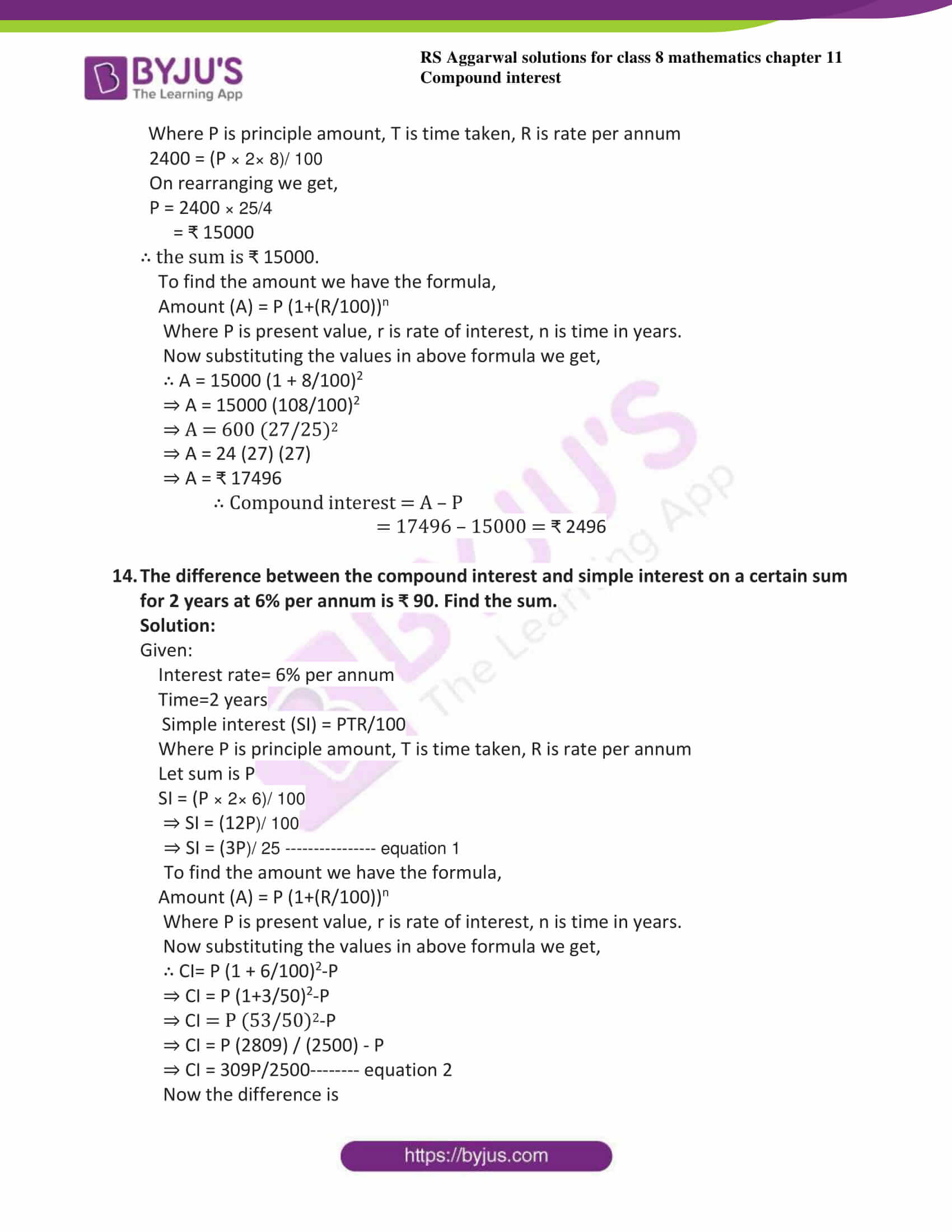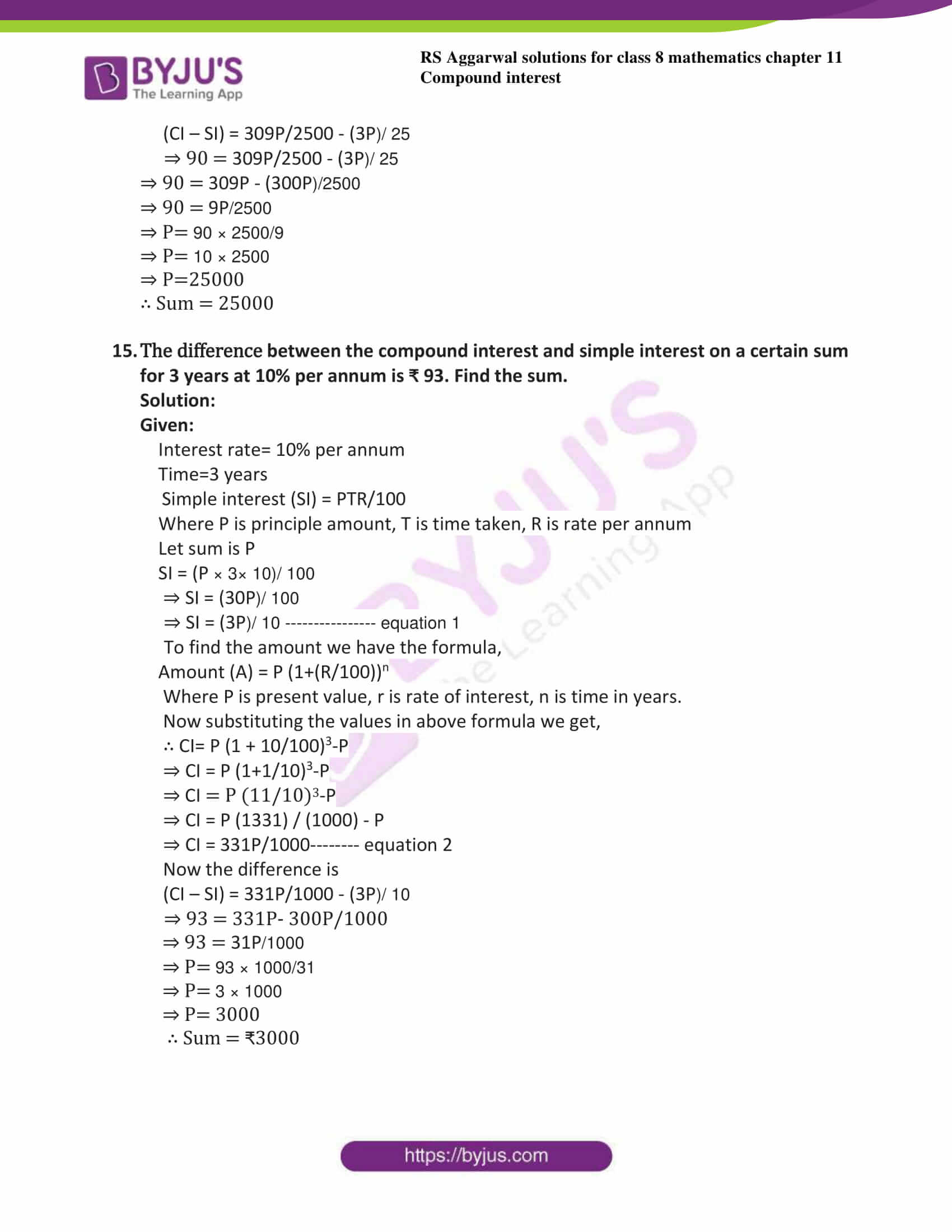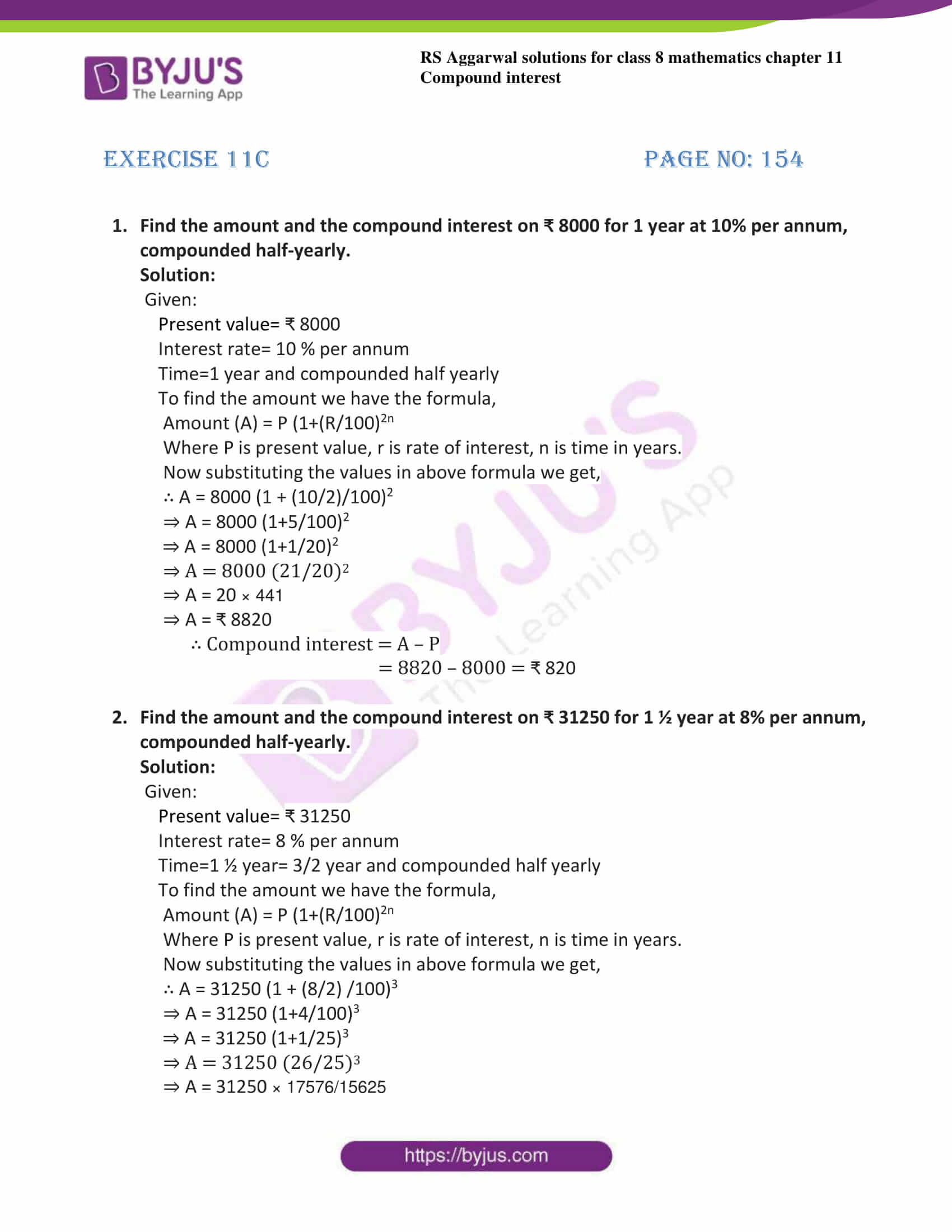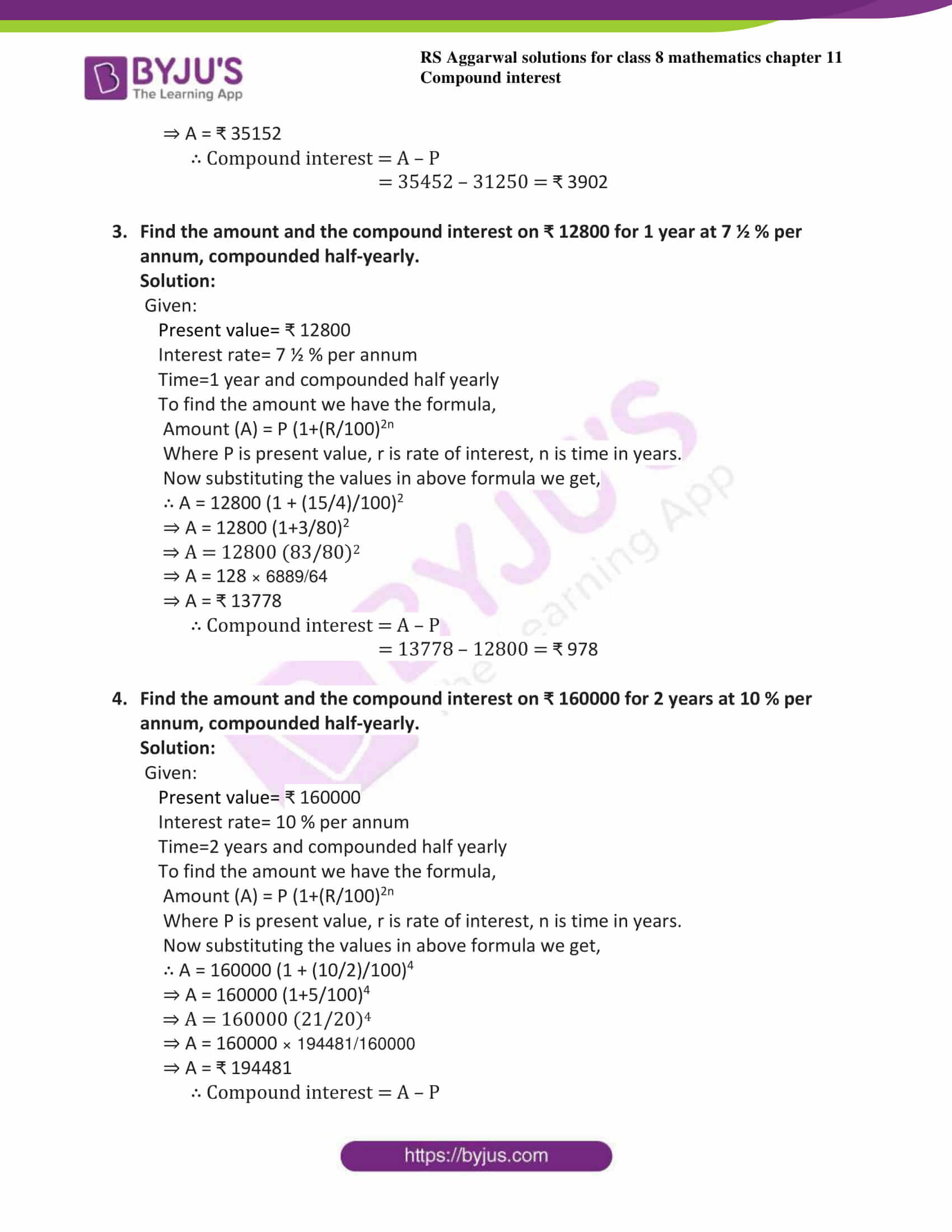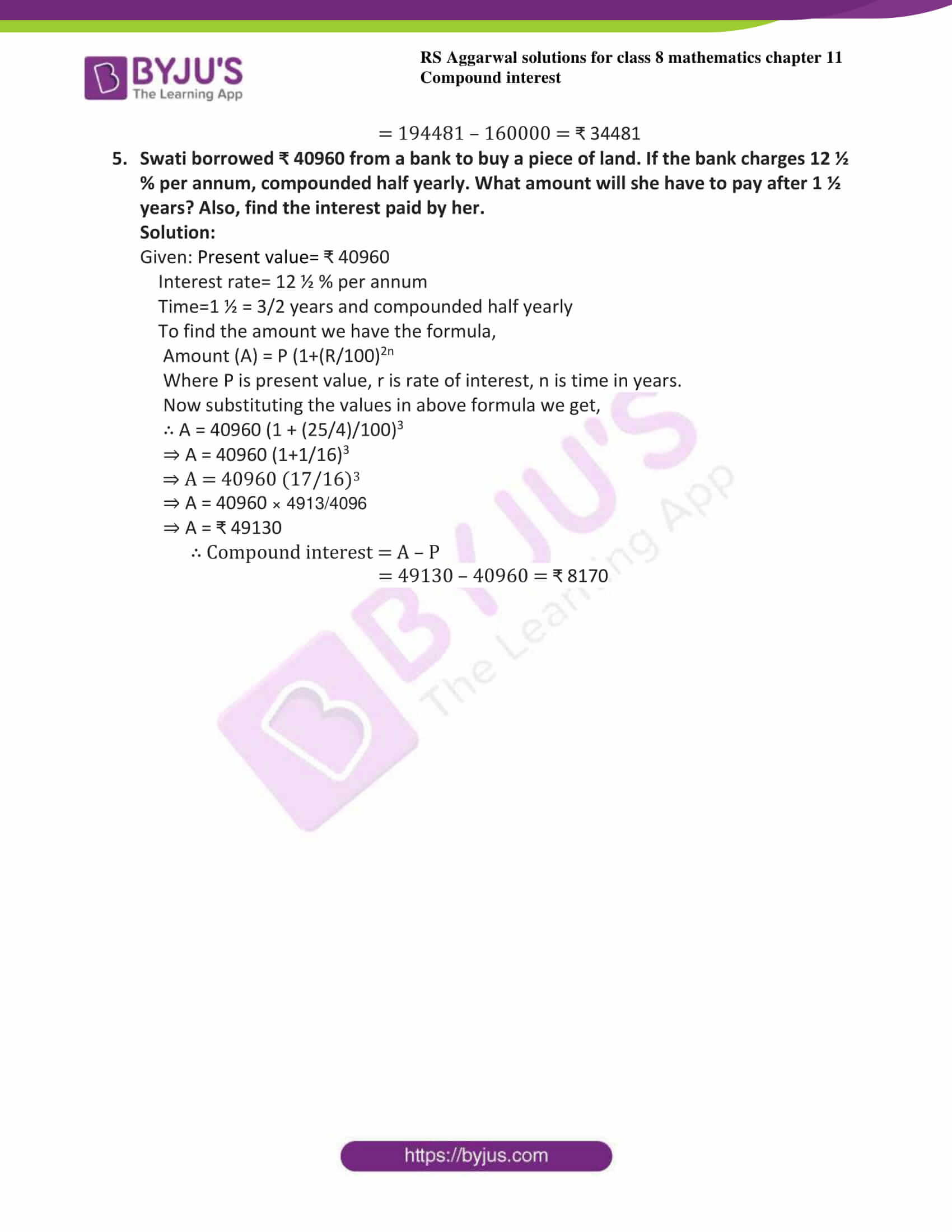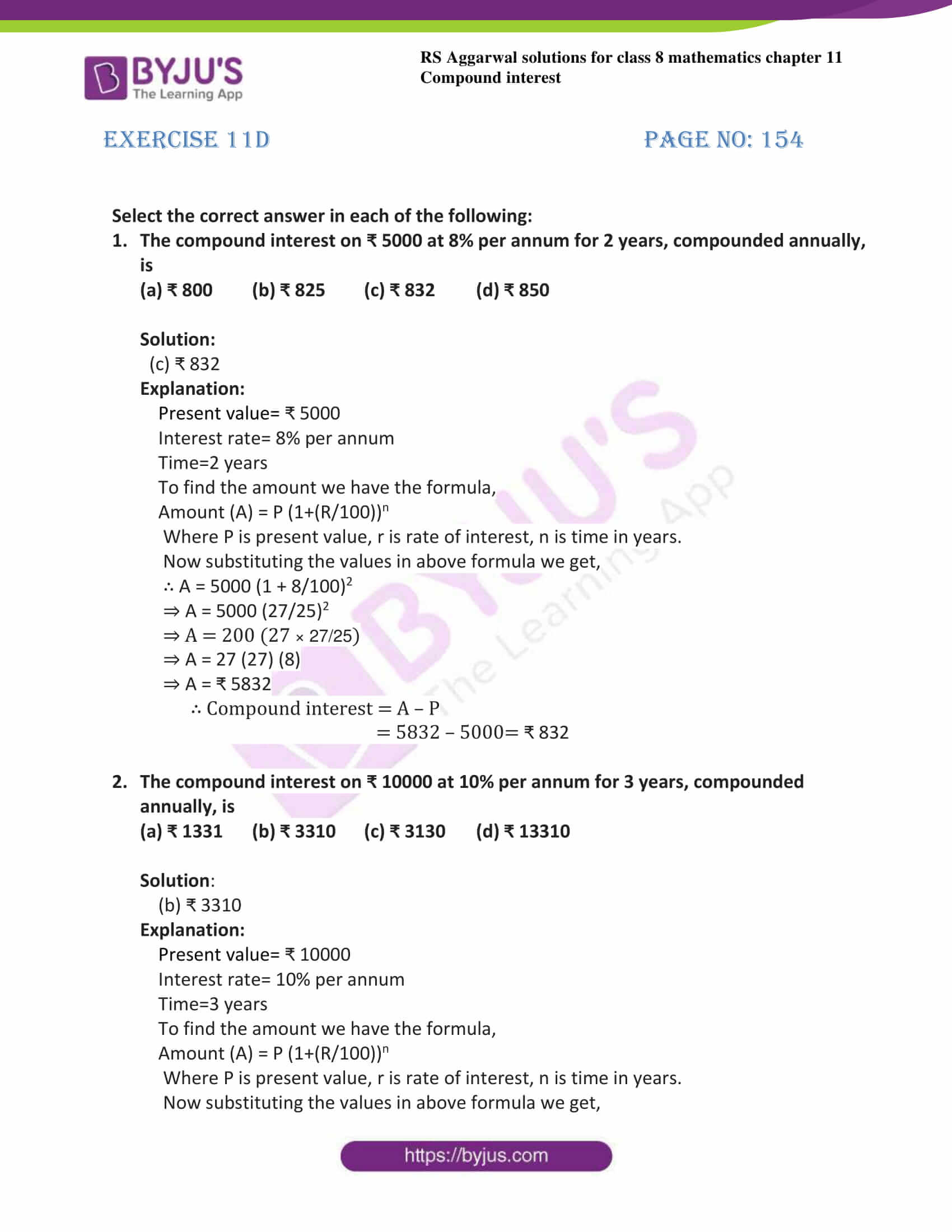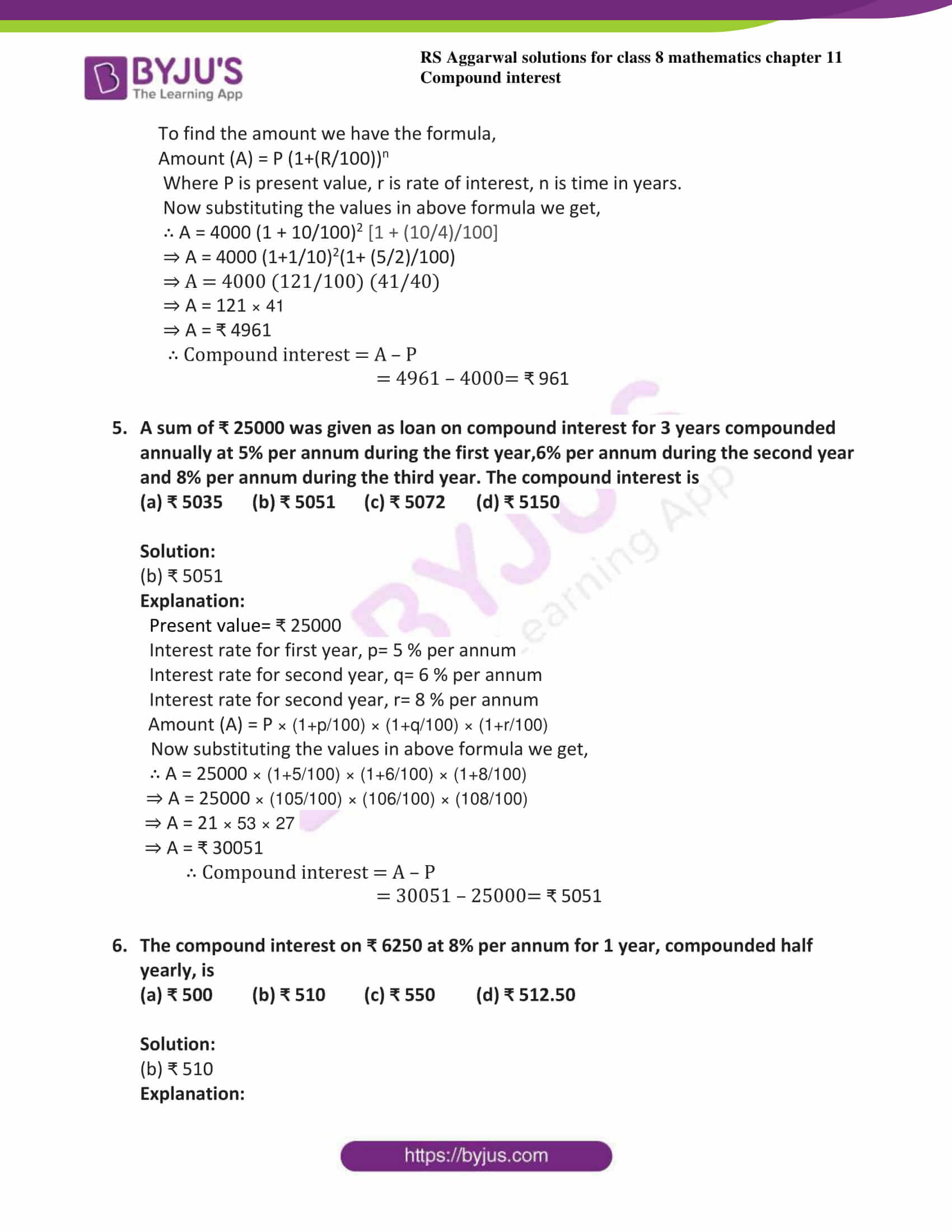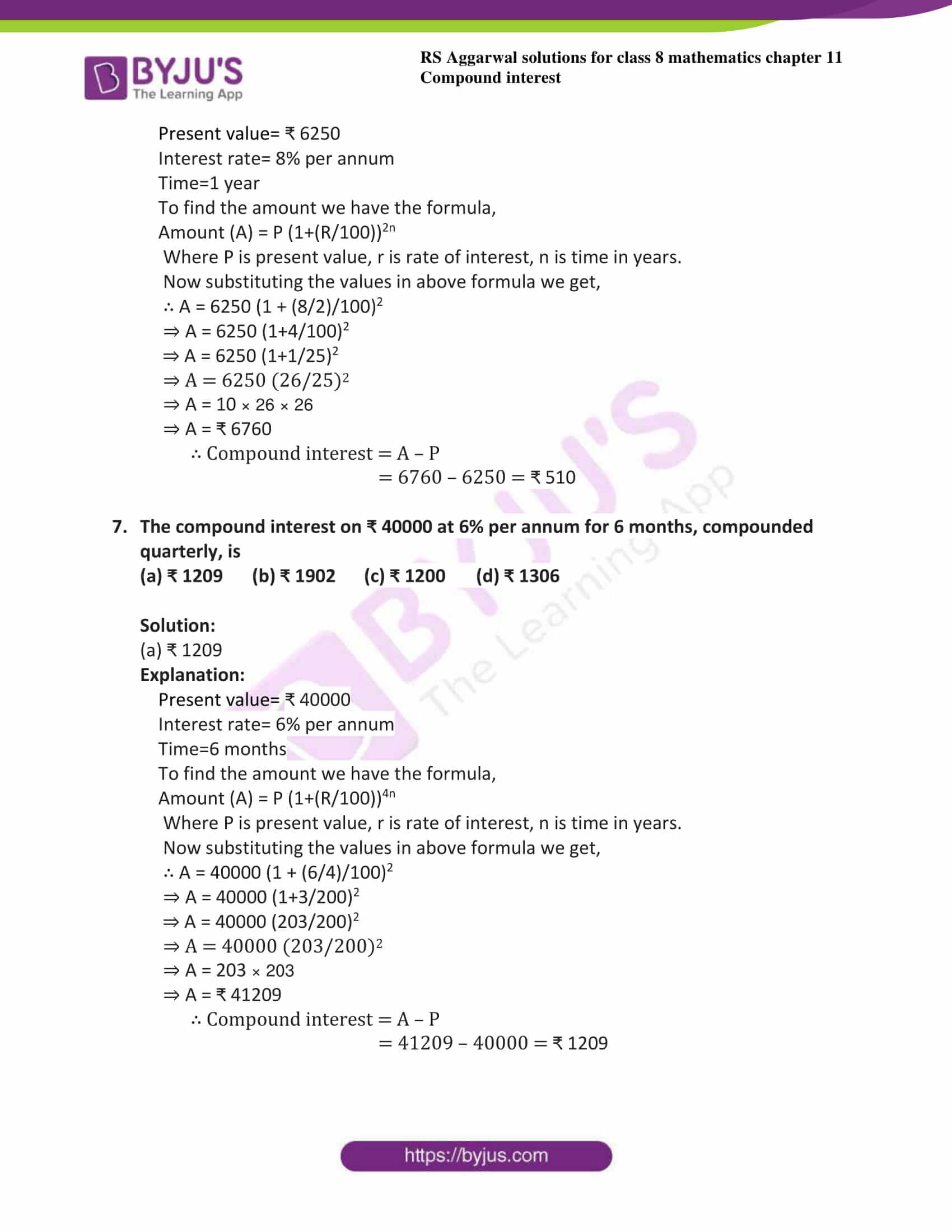## Exercise 11A

1. Find the amount and the compound interest on ₹ 2500 for 2 years at 10% per annum, compounded annually.

Solution:

Given:

Present value= ₹ 2500

Interest rate= 10% per annum

Time=2 years

To find the amount we have the formula,

Amount (A) = P (1+(R/100))n

Where P is present value, r is rate of interest, n is time in years.

Now substituting the values in above formula we get,

∴ A = 2500 (1 + 10/100)2

⇒ A = 2500 (11/10)2

⇒ A = 2500 (121/100)

⇒ A = 25 (121)

⇒ A = ₹ 3025

∴ Compound interest = A – P

= 3025 – 2500= ₹ 525

2. Find the amount and the compound interest on ₹ 15625 for 3 years at 12% per annum, compounded annually.

Solution:

Given:

Present value= ₹ 15625

Interest rate= 12% per annum

Time=3 years

To find the amount we have the formula,

Amount (A) = P (1+(R/100))n

Where P is present value, r is rate of interest, n is time in years.

Now substituting the values in above formula we get,

∴ A = 15625 (1 + 12/100)3

⇒ A = 15625 (112/100)3

⇒ A = 15625 (28/25)3

⇒ A = 15625 (21952/15625)

⇒ A = ₹ 21925

∴ Compound interest = A – P

= 21925 – 15625 = ₹ 6327

3. Find the difference between the simple interest and the compound interest on ₹ 5000 for 2 years at 9% per annum.

Solution:

Given:

Present value= ₹ 5000

Interest rate= 9% per annum

Time=2 years

Now find compound interest,

To find the amount we have the formula,

Amount (A) = P (1+(R/100))n

Where P is present value, r is rate of interest, n is time in years.

Now substituting the values in above formula we get,

∴ A = 5000 (1 + 9/100)2

⇒ A = 5000 (109/100)2

⇒ A = 5000 (1.09)2

⇒ A = 5000 (1.1881)

⇒ A = ₹ 5940.5

∴ Compound interest = A – P

= 5940.5 – 5000 = ₹ 940.5

Now find the simple interest,

Simple interest (SI) = PTR/100

Where P is principle amount, T is time taken, R is rate per annum

SI = (5000 × 2 × 9) / 100

= 50 × 2 × 9

= ₹ 900

Difference between compound interest and simple interest is = (CI – SI)

= 940.5 – 900

= ₹ 40.5

4. Ratna obtained a loan of ₹ 25000 from the Syndicate bank to renovate her house. If the rate of interest is 8% per annum. What amount will she have to pay to the bank after 2 years to discharge her debt?

Solution:

Given:

Present value= ₹ 25000

Interest rate= 8% per annum

Time=2 years

To find the amount we have the formula,

Amount (A) = P (1+(R/100))n

Where P is present value, r is rate of interest, n is time in years.

Now substituting the values in above formula we get,

∴ A = 25000 (1 + 8/100)2

⇒ A = 25000 (108/100)2

⇒ A = 25000 (1.08)2

⇒ A = 25000(1.1664)

⇒ A = ₹ 29160

Ratna has to pay ₹ 29160

## Exercise 11B

By using the formula, find the amount and compound interest on:

1. ₹ 6000 for 2 years at 9% per annum compounded annually.

Solution:

Given:

Present value= ₹ 6000

Interest rate= 9% per annum

Time=2 years

To find the amount we have the formula,

Amount (A) = P (1+(R/100))n

Where P is present value, r is rate of interest, n is time in years.

Now substituting the values in above formula we get,

∴ A = 6000 (1 + 9/100)2

⇒ A = 6000 (109/100)2

⇒ A = 6000 (1.09)2

⇒ A = 7128.6

⇒ A = ₹ 7128.6

∴ Compound interest = A – P

= 7128.6 – 6000= ₹ 1128.6

2. ₹ 10000 for 2 years at 11% per annum compounded annually.

Solution:

Given:

Present value= ₹ 10000

Interest rate= 11% per annum

Time=2 years

To find the amount we have the formula,

Amount (A) = P (1+(R/100))n

Where P is present value, r is rate of interest, n is time in years.

Now substituting the values in above formula we get,

∴ A = 10000 (1 + 11/100)2

⇒ A = 10000 (111/100)2

⇒ A = 10000 (111/100) (111/100)

⇒ A = 111 × 111

⇒ A = ₹ 12321

∴ Compound interest = A – P

= 12321 – 10000= ₹ 2321

3. ₹ 31250 for 3 years at 8% per annum compounded annually.

Solution:

Given:

Present value= ₹ 31250

Interest rate= 8% per annum

Time=3 years

To find the amount we have the formula,

Amount (A) = P (1+(R/100))n

Where P is present value, r is rate of interest, n is time in years.

Now substituting the values in above formula we get,

∴ A = 31250 (1 + 8/100)3

⇒ A = 31250 (1+2/25)3

⇒ A = 31250 (27/25)3

⇒ A = 31250 × 19683/15625 = 2 × 19683

⇒ A = ₹ 39366

∴ Compound interest = A – P

= 39366 – 31250= ₹ 8116

4. ₹ 10240 for 3 years at 12 ½ % per annum compounded annually.

Solution:

Given:

Present value= ₹ 10240

Interest rate= 12 ½ % per annum = 25/2%

Time=3 years

To find the amount we have the formula,

Amount (A) = P (1+(R/100))n

Where P is present value, r is rate of interest, n is time in years.

Now substituting the values in above formula we get,

∴ A = 10240 (1 + (25/2)/100)3

⇒ A = 10240 (1+1/8)3

⇒ A = 10240 (9/8)3

⇒ A = 31250 × 729/512 = 20 × 729

⇒ A = ₹ 14580

∴ Compound interest = A – P

= 14580 – 10240= ₹ 4340

5. ₹ 62500 for 2 years 6 months at 12 % per annum compounded annually.

Solution:

Given:

Present value= ₹ 62500

Interest rate= 12 % per annum

Time=2 years 6 months = (2 + ½) years= 5/2 years

To find the amount we have the formula,

Amount (A) = P (1+(R/100))n

Where P is present value, r is rate of interest, n is time in years.

Now substituting the values in above formula we get,

∴ A = 62500 (1 + 12/100)2 [1 + (1/2 × 12)/100]

⇒ A = 62500 (1+3/25)2 (1+6/100)

⇒ A = 62500 (28/25)2 (106/100)

⇒ A = 625 × 784/625 × 106 = 784 × 106

⇒ A = ₹ 83104

∴ Compound interest = A – P

= 83104 – 62500 = ₹ 20604

6. ₹ 9000 for 2 years 4 months at 10 % per annum compounded annually.

Solution:

Given:

Present value= ₹ 9000

Interest rate= 10 % per annum

Time=2 years 6 months = (2 + ½) years= 5/2 years

To find the amount we have the formula,

Amount (A) = P (1+(R/100))n

Where P is present value, r is rate of interest, n is time in years.

Now substituting the values in above formula we get,

∴ A = 9000 (1 + 10/100)2 [1 + (1/3 × 10)/100]

⇒ A = 9000 (1+1/10)2 (1+1/30)

⇒ A = 9000 (11/10)2 (31/30)

⇒ A = 9000 × 121/100 × 31/30 = 9 × 121 × 31/3

⇒ A = ₹ 11253

∴ Compound interest = A – P

= 11253 – 6000 = ₹ 2253

7. Find the amount of ₹ 8000 for 2 years compounded annually and the rates being 9% per annum during the first year and 10% per annum during the second year.

Solution:

Given:

Present value= ₹ 8000

Interest rate for first year, p= 9 % per annum

Interest rate for second year, q= 10 % per annum

Amount (A) = P × (1+p/100) × (1+q/100)

Now substituting the values in above formula we get,

∴ A = 8000 × (1+9/100) × (1+10/100)

⇒ A = 8000 × (109/100) × (11/10)

⇒ A = 8 × 109 × 11

⇒ A = ₹ 9592

8. Anand obtained a loan of ₹ 125000 from the Allahabad bank for buying computers. The bank charges compound interest at 8% per annum, compounded annually. What amount will have to pay after 3 years to clear the debt?

Solution:

Given:

Present value= ₹ 125000

Interest rate= 8 % per annum

Time= 3 years

To find the amount we have the formula,

Amount (A) = P (1+(R/100))n

Where P is present value, r is rate of interest, n is time in years.

Now substituting the values in above formula we get,

∴ A = 125000 (1 + 8/100)3

⇒ A = 125000 (1+108/100)3

⇒ A = 125000 (108/100) (108/100) (108/100)

⇒ A = 125000 × 1259712/1000 = 1259712/8

⇒ A = ₹ 157464

Anand has to pay ₹ 157464

9. Three years ago, Beeru purchased a buffalo from Surjeet for ₹ 11000. What payment

Will discharge his debt now, the rate of interest being 10% per annum, compounded annually?

Solution:

Given:

Present value= ₹ 11000

Interest rate= 10% per annum

Time=3 years

To find the amount we have the formula,

Amount (A) = P (1+(R/100))n

Where P is present value, r is rate of interest, n is time in years.

Now substituting the values in above formula we get,

∴ A = 11000 (1 + 10/100)3

⇒ A = 11000 (11/10)3

⇒ A = 11000 (1331/1000)

⇒ A = 11 (1331)

⇒ A = ₹ 14614

∴ Beeru has to pay ₹ 14614

10. Shubhalaxmi took a loan of ₹ 18000 from Surya Finance to purchase a TV set. If the company charges compound interest at 12% per annum during the first year and 12 ½ % per annum during the second year, how much will she have to pay after 2 years?

Solution:

Given:

Present value= ₹ 18000

Interest rate for first year, p= 12 % per annum

Interest rate for second year, q= 12 ½ % per annum

Amount (A) = P × (1+p/100) × (1+q/100)

Now substituting the values in above formula we get,

∴ A = 18000 × (1+12/100) × (1 + (25/2)/100)

⇒ A = 8000 × (112/100) × (1+25/200)

⇒ A = 8000 × (112/100) × (1+1/8)

⇒ A = 180 × 14 × 8

⇒ A = ₹ 22680

Shubhalaxmi has to pay ₹ 22680

11. Neha borrowed ₹ 24000 from the state bank of India to buy a scooter. If the rate of interest be 10% per annum compounded annually, what payment will she have to make after 2 years 3 months?

Solution:

Given:

Present value= ₹ 24000

Interest rate= 10 % per annum

Time= 2 years 3 months = 2 ¼ years

To find the amount we have the formula,

Amount (A) = P (1+(R/100))n

Where P is present value, r is rate of interest, n is time in years.

Now substituting the values in above formula we get,

∴ A = 24000 (1 + 10/100)2 [1 + (1/4 × 10)/100]

⇒ A = 24000 (1+1/10)2 (1+1/40)

⇒ A = 24000 (11/10)2 (41/40)

⇒ A = 24000 × 121/100 × 41/40 = 24 × 121 × 41/4

⇒ A = ₹ 29766

∴ Neha has to pay ₹ 29766

12. Abhay borrowed ₹ 16000 at 7 ½ % per annum simple interest. On the same day, he lent it to gurmeet at the same rate but compounded annually. What does he gain at the end of 2 years?

Solution:

Given:

Present value= ₹ 16000

Interest rate= 7 ½ % per annum= 15/2 %

Time=2 years

Now find compound interest,

To find the amount we have the formula,

Amount (A) = P (1+(R/100))n

Where P is present value, r is rate of interest, n is time in years.

Now substituting the values in above formula we get,

∴ A = 16000 (1 + (15/2)/100)2

⇒ A = 16000 (1+3/40)2

⇒ A =16000 (43/40)2

⇒ A = 16000 (1894/1600)

⇒ A = ₹ 18490

∴ Compound interest = A – P

= 18490 – 16000 = ₹ 2490

Now find the simple interest,

Simple interest (SI) = PTR/100

Where P is principle amount, T is time taken, R is rate per annum

SI = (16000 × (15/2) × 2) / 100

= 160 × 15

= ₹ 2400

Abhay gains at the end of 2 year= (CI – SI)

= 2490 – 2400

= ₹ 90

13. The simple interest on a sum of money for 2 years at 8% per annum is ₹ 2400. What will be the compound interest on that sum at the rate and for the same period?

Solution:

Given:

Simple interest= ₹ 2400

Interest rate= 8% per annum

Time=2 years

Simple interest (SI) = PTR/100

Where P is principle amount, T is time taken, R is rate per annum

2400 = (P × 2× 8)/ 100

On rearranging we get,

P = 2400 × 25/4

= ₹ 15000

∴ the sum is ₹ 15000.

To find the amount we have the formula,

Amount (A) = P (1+(R/100))n

Where P is present value, r is rate of interest, n is time in years.

Now substituting the values in above formula we get,

∴ A = 15000 (1 + 8/100)2

⇒ A = 15000 (108/100)2

⇒ A = 600 (27/25)2

⇒ A = 24 (27) (27)

⇒ A = ₹ 17496

∴ Compound interest = A – P

= 17496 – 15000 = ₹ 2496

14. The difference between the compound interest and simple interest on a certain sum for 2 years at 6% per annum is ₹ 90. Find the sum.

Solution:

Given:

Interest rate= 6% per annum

Time=2 years

Simple interest (SI) = PTR/100

Where P is principle amount, T is time taken, R is rate per annum

Let sum is P

SI = (P × 2× 6)/ 100

⇒ SI = (12P)/ 100

⇒ SI = (3P)/ 25 —————- equation 1

To find the amount we have the formula,

Amount (A) = P (1+(R/100))n

Where P is present value, r is rate of interest, n is time in years.

Now substituting the values in above formula we get,

∴ CI= P (1 + 6/100)2-P

⇒ CI = P (1+3/50)2-P

⇒ CI = P (53/50)2-P

⇒ CI = P (2809) / (2500) – P

⇒ CI = 309P/2500——– equation 2

Now the difference is

(CI – SI) = 309P/2500 – (3P)/ 25

⇒ 90 = 309P/2500 – (3P)/ 25

⇒ 90 = 309P – (300P)/2500

⇒ 90 = 9P/2500

⇒ P= 90 × 2500/9

⇒ P= 10 × 2500

⇒ P=25000

∴ Sum = 25000

15. The difference between the compound interest and simple interest on a certain sum for 3 years at 10% per annum is ₹ 93. Find the sum.

Solution:

Given:

Interest rate= 10% per annum

Time=3 years

Simple interest (SI) = PTR/100

Where P is principle amount, T is time taken, R is rate per annum

Let sum is P

SI = (P × 3× 10)/ 100

⇒ SI = (30P)/ 100

⇒ SI = (3P)/ 10 —————- equation 1

To find the amount we have the formula,

Amount (A) = P (1+(R/100))n

Where P is present value, r is rate of interest, n is time in years.

Now substituting the values in above formula we get,

∴ CI= P (1 + 10/100)3-P

⇒ CI = P (1+1/10)3-P

⇒ CI = P (11/10)3-P

⇒ CI = P (1331) / (1000) – P

⇒ CI = 331P/1000——– equation 2

Now the difference is

(CI – SI) = 331P/1000 – (3P)/ 10

⇒ 93 = 331P- 300P/1000

⇒ 93 = 31P/1000

⇒ P= 93 × 1000/31

⇒ P= 3 × 1000

⇒ P= 3000

∴ Sum = ₹3000

## Exercise 11C

1. Find the amount and the compound interest on ₹ 8000 for 1 year at 10% per annum, compounded half-yearly.

Solution:

Given:

Present value= ₹ 8000

Interest rate= 10 % per annum

Time=1 year and compounded half yearly

To find the amount we have the formula,

Amount (A) = P (1+(R/100)2n

Where P is present value, r is rate of interest, n is time in years.

Now substituting the values in above formula we get,

∴ A = 8000 (1 + (10/2)/100)2

⇒ A = 8000 (1+5/100)2

⇒ A = 8000 (1+1/20)2

⇒ A = 8000 (21/20)2

⇒ A = 20 × 441

⇒ A = ₹ 8820

∴ Compound interest = A – P

= 8820 – 8000 = ₹ 820

2. Find the amount and the compound interest on ₹ 31250 for 1 ½ year at 8% per annum, compounded half-yearly.

Solution:

Given:

Present value= ₹ 31250

Interest rate= 8 % per annum

Time=1 ½ year= 3/2 year and compounded half yearly

To find the amount we have the formula,

Amount (A) = P (1+(R/100)2n

Where P is present value, r is rate of interest, n is time in years.

Now substituting the values in above formula we get,

∴ A = 31250 (1 + (8/2) /100)3

⇒ A = 31250 (1+4/100)3

⇒ A = 31250 (1+1/25)3

⇒ A = 31250 (26/25)3

⇒ A = 31250 × 17576/15625

⇒ A = ₹ 35152

∴ Compound interest = A – P

= 35452 – 31250 = ₹ 3902

3. Find the amount and the compound interest on ₹ 12800 for 1 year at 7 ½ % per annum, compounded half-yearly.

Solution:

Given:

Present value= ₹ 12800

Interest rate= 7 ½ % per annum

Time=1 year and compounded half yearly

To find the amount we have the formula,

Amount (A) = P (1+(R/100)2n

Where P is present value, r is rate of interest, n is time in years.

Now substituting the values in above formula we get,

∴ A = 12800 (1 + (15/4)/100)2

⇒ A = 12800 (1+3/80)2

⇒ A = 12800 (83/80)2

⇒ A = 128 × 6889/64

⇒ A = ₹ 13778

∴ Compound interest = A – P

= 13778 – 12800 = ₹ 978

4. Find the amount and the compound interest on ₹ 160000 for 2 years at 10 % per annum, compounded half-yearly.

Solution:

Given:

Present value= ₹ 160000

Interest rate= 10 % per annum

Time=2 years and compounded half yearly

To find the amount we have the formula,

Amount (A) = P (1+(R/100)2n

Where P is present value, r is rate of interest, n is time in years.

Now substituting the values in above formula we get,

∴ A = 160000 (1 + (10/2)/100)4

⇒ A = 160000 (1+5/100)4

⇒ A = 160000 (21/20)4

⇒ A = 160000 × 194481/160000

⇒ A = ₹ 194481

∴ Compound interest = A – P

= 194481 – 160000 = ₹ 34481

5. Swati borrowed ₹ 40960 from a bank to buy a piece of land. If the bank charges 12 ½ % per annum, compounded half yearly. What amount will she have to pay after 1 ½ years? Also, find the interest paid by her.

Solution:

Given: Present value= ₹ 40960

Interest rate= 12 ½ % per annum

Time=1 ½ = 3/2 years and compounded half yearly

To find the amount we have the formula,

Amount (A) = P (1+(R/100)2n

Where P is present value, r is rate of interest, n is time in years.

Now substituting the values in above formula we get,

∴ A = 40960 (1 + (25/4)/100)3

⇒ A = 40960 (1+1/16)3

⇒ A = 40960 (17/16)3

⇒ A = 40960 × 4913/4096

⇒ A = ₹ 49130

∴ Compound interest = A – P

= 49130 – 40960 = ₹ 8170

## Exercise 11D Page No: 154

Select the correct answer in each of the following:

1. The compound interest on ₹ 5000 at 8% per annum for 2 years, compounded annually, is

(a) ₹ 800 (b) ₹ 825 (c) ₹ 832 (d) ₹ 850

Solution:

(c) ₹ 832

Explanation:

Present value= ₹ 5000

Interest rate= 8% per annum

Time=2 years

To find the amount we have the formula,

Amount (A) = P (1+(R/100))n

Where P is present value, r is rate of interest, n is time in years.

Now substituting the values in above formula we get,

∴ A = 5000 (1 + 8/100)2

⇒ A = 5000 (27/25)2

⇒ A = 200 (27 × 27/25)

⇒ A = 27 (27) (8)

⇒ A = ₹ 5832

∴ Compound interest = A – P

= 5832 – 5000= ₹ 832

2. The compound interest on ₹ 10000 at 10% per annum for 3 years, compounded annually, is

(a) ₹ 1331 (b) ₹ 3310 (c) ₹ 3130 (d) ₹ 13310

Solution:

(b) ₹ 3310

Explanation:

Present value= ₹ 10000

Interest rate= 10% per annum

Time=3 years

To find the amount we have the formula,

Amount (A) = P (1+(R/100))n

Where P is present value, r is rate of interest, n is time in years.

Now substituting the values in above formula we get,

∴ A = 10000 (1 + 10/100)3

⇒ A = 10000 (11/10)3

⇒ A = 121 (10) (11)

⇒ A = 1331 (10)

⇒ A = ₹ 13310

∴ Compound interest = A – P

= 13310 – 10000= ₹ 3310

3. The compound interest on ₹ 10000 at 12% per annum for 1 ½ years, compounded annually, is

(a) ₹ 1872 (b) ₹ 1720 (c) ₹ 1910.16 (d) ₹ 1782

Solution:

(a) ₹ 1872

Explanation:

Present value= ₹ 10000

Interest rate= 12% per annum

Time=1 ½ years

To find the amount we have the formula,

Amount (A) = P (1+(R/100))n

Where P is present value, r is rate of interest, n is time in years.

Now substituting the values in above formula we get,

∴ A = 10000 (1 + 12/100)1 [1 + (12/2 )/100]

⇒ A = 10000 (1+12/100)1(1+6/100)

⇒ A = 10000 (112/100) (106/100)

⇒ A = 1 × 112 × 106

⇒ A = ₹ 11872

∴ Compound interest = A – P

= 11872 – 10000= ₹ 1872

4. The compound interest on ₹ 4000 at 10% per annum for 2 years 3 months , compounded annually, is

(a) ₹ 916 (b) ₹ 900 (c) ₹ 961 (d) ₹ 896

Solution:

(c) ₹ 961

Explanation:

Present value= ₹ 4000

Interest rate= 10% per annum

Time=2 ¼ years

To find the amount we have the formula,

Amount (A) = P (1+(R/100))n

Where P is present value, r is rate of interest, n is time in years.

Now substituting the values in above formula we get,

∴ A = 4000 (1 + 10/100)2 [1 + (10/4)/100]

⇒ A = 4000 (1+1/10)2(1+ (5/2)/100)

⇒ A = 4000 (121/100) (41/40)

⇒ A = 121 × 41

⇒ A = ₹ 4961

∴ Compound interest = A – P

= 4961 – 4000= ₹ 961

5. A sum of ₹ 25000 was given as loan on compound interest for 3 years compounded annually at 5% per annum during the first year,6% per annum during the second year and 8% per annum during the third year. The compound interest is

(a) ₹ 5035 (b) ₹ 5051 (c) ₹ 5072 (d) ₹ 5150

Solution:

(b) ₹ 5051

Explanation:

Present value= ₹ 25000

Interest rate for first year, p= 5 % per annum

Interest rate for second year, q= 6 % per annum

Interest rate for second year, r= 8 % per annum

Amount (A) = P × (1+p/100) × (1+q/100) × (1+r/100)

Now substituting the values in above formula we get,

∴ A = 25000 × (1+5/100) × (1+6/100) × (1+8/100)

⇒ A = 25000 × (105/100) × (106/100) × (108/100)

⇒ A = 21 × 53 × 27

⇒ A = ₹ 30051

∴ Compound interest = A – P

= 30051 – 25000= ₹ 5051

6. The compound interest on ₹ 6250 at 8% per annum for 1 year, compounded half yearly, is

(a) ₹ 500 (b) ₹ 510 (c) ₹ 550 (d) ₹ 512.50

Solution:

(b) ₹ 510

Explanation:

Present value= ₹ 6250

Interest rate= 8% per annum

Time=1 year

To find the amount we have the formula,

Amount (A) = P (1+(R/100))2n

Where P is present value, r is rate of interest, n is time in years.

Now substituting the values in above formula we get,

∴ A = 6250 (1 + (8/2)/100)2

⇒ A = 6250 (1+4/100)2

⇒ A = 6250 (1+1/25)2

⇒ A = 6250 (26/25)2

⇒ A = 10 × 26 × 26

⇒ A = ₹ 6760

∴ Compound interest = A – P

= 6760 – 6250 = ₹ 510

7. The compound interest on ₹ 40000 at 6% per annum for 6 months, compounded quarterly, is

(a) ₹ 1209 (b) ₹ 1902 (c) ₹ 1200 (d) ₹ 1306

Solution:

(a) ₹ 1209

Explanation:

Present value= ₹ 40000

Interest rate= 6% per annum

Time=6 months

To find the amount we have the formula,

Amount (A) = P (1+(R/100))4n

Where P is present value, r is rate of interest, n is time in years.

Now substituting the values in above formula we get,

∴ A = 40000 (1 + (6/4)/100)2

⇒ A = 40000 (1+3/200)2

⇒ A = 40000 (203/200)2

⇒ A = 40000 (203/200)2

⇒ A = 203 × 203

⇒ A = ₹ 41209

∴ Compound interest = A – P

= 41209 – 40000 = ₹ 1209

## RS Aggarwal Solutions for Class 8 Maths Chapter 11- Compound Interest

Chapter 11, Compound Interest, contains 4 Exercises. RS Aggarwal Solutions given here contains the answers to all the questions present in these exercises. Let us have a look at some of the concepts that are being discussed in this chapter.

• Introduction to compound interest
• Finding the Compound interest when interest is compounded annually
• Finding the compound interest when interest is compounded half-yearly
• Calculating compound interest by using formulae
• When the interest is compounded annually
• When the interest is compounded annually but rates are different for different years
• When interest is compounded annually but time is a fraction
• Applications of compound interest formula

Exercise 11A

Exercise 11B

Exercise 11C

Exercise 11D

### Chapter Brief of RS Aggarwal Solutions for Class 8 Maths Chapter 11 – Compound Interest

The RS Aggarwal Solutions for Class 8 Maths Chapter 11 – Compound Interest deals with the definition of compound interest, finding the compound interest when interest is compounded annually and half-yearly using the formulae and without using the formulae. It also deals with applications of compound interest.